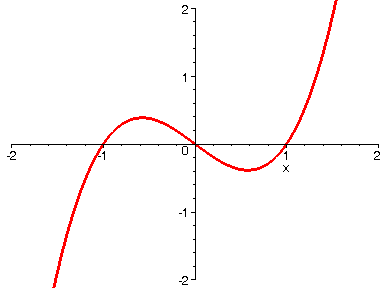VERTICAL COMPRESSION

If you take a function y = f(x) and replace it by y = k*f(x) where 0 < k < 1, then the net result is that the graph of f(x) is compressed vertically toward the horizontal axis. Below we start with a polynomial function of the form y = k*f(x), and the animation shows the effect on the graph as we let k decrease from 1 to 1/20. By the way, this effect looks similar to that of a horizontal stretch. How can we tell the difference?

> with(plots):

> f:=x->x^3-1*x;> A := seq(plot((1/i)*f(x), x=-5..5, numpoints=500, thickness=3), i=1..20):

> display(A, insequence=true, view=[-2..2, -2..2]);>